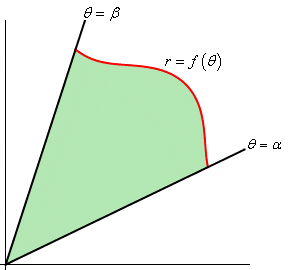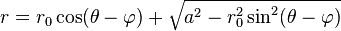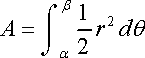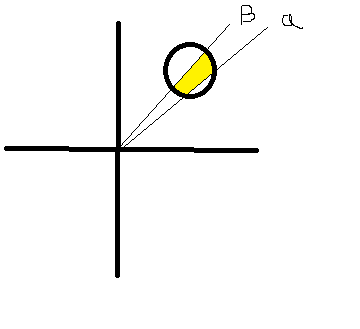# Area of a Circle in Polar Coordinates

dluu
Hi,

I'm trying to find the area of a segment of a circle that is not at the origin. It will look similar to this picture below but I need to find the area enclosed by a circle.Using the polar equation of a circle provided by wikipedia:and integrating to find the area of a curve:Resulting in:

http://desmond.imageshack.us/Himg145/scaled.php?server=145&filename=equation.png&res=landing [Broken]

Am I on the right track or is there a more simple way? I'm not sure how to integrate this kind of function. I tried and got so lost.

Last edited by a moderator:

dluu
I think I went the wrong way with this problem.

Here is a better picture:I am trying to find find a general equation to plug into Excel. Depending on location of the circle, radius, and limits of integration, will give a value of the area.

I'm not sure using the polar equation of a circle in the original post is the best way to go at this. Will using the cartesian form of a circle (x-h)^2 - (y-k)^2 = r^2 and converting to polar form work? I tried to do this which gave a huge equation to integrate. I worked it out but when I tried to prove the equation, it failed. Can anyone help?

lugita15
I think the simplest way to do this is to define a new coordinate system with the origin at the center of your circle. That way the equation of the circle is just r=R where R is the radius of the circle.

Homework Helper
I think the simplest way to do this is to define a new coordinate system with the origin at the center of your circle. That way the equation of the circle is just r=R where R is the radius of the circle.

I don't think this will simplify the problem. The region of the circle that the OP wants to integrate over is not at all simple when the circle is at the origin. It looks like you would have to introduce a radial integration in addition to an angular integration in order to get the desired wedge of the circle, and at least one set of limits would be complicated.

To the OP: I'm not sure if that expression can be completely written down in a closed form, but you can at least write down pieces of it in a closed from and then you may have to write some routine to approximately compute the integrals you can't evaluate in closed form.

You can write ##\cos^2(\theta-\phi) - \sin^2(\theta-\phi) = \cos(2(\theta-\phi))##, which you can integrate, and then you have some constant terms which are easy to integrate, so it looks like it's the

$$\cos(\theta-\phi)\sqrt{R^2-r_0^2\sin^2(\theta-\phi)}$$

term that you may have to evaluate separately, perhaps using a trapezoid-rule like algorithm to approximate the integral numerically for specified parameters.

Edit: Actually, you can do that integral too, although it can get tricky because you have to be careful about quadrants. Basically, you can let ##x = \sin(\theta-\phi)##, in which case ##dx = cos(\theta-\phi)d\theta##, but if your limits are chosen such that ##\sin(\theta-\phi)## is not monotonic over the integration region, you have to split the integral up into separate pieces on which ##\sin(\theta-\phi)## is monotonic before you can make that change of variables.

Last edited: